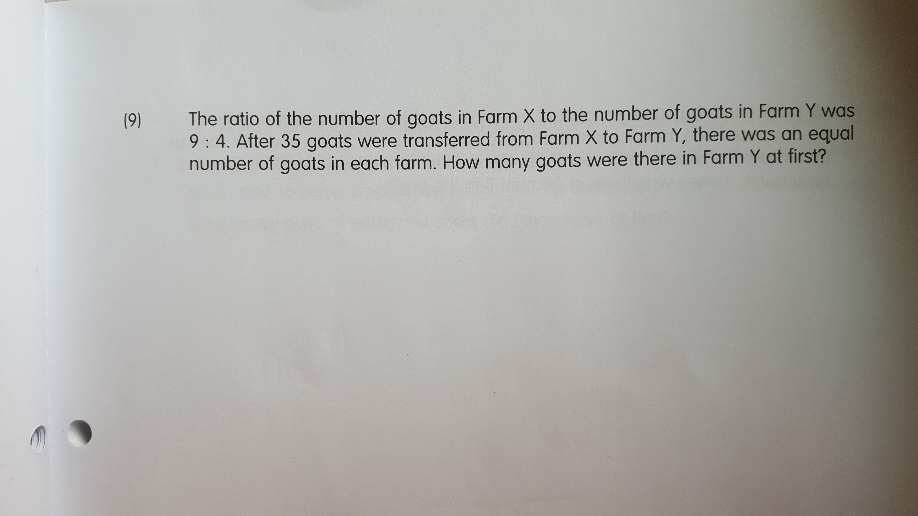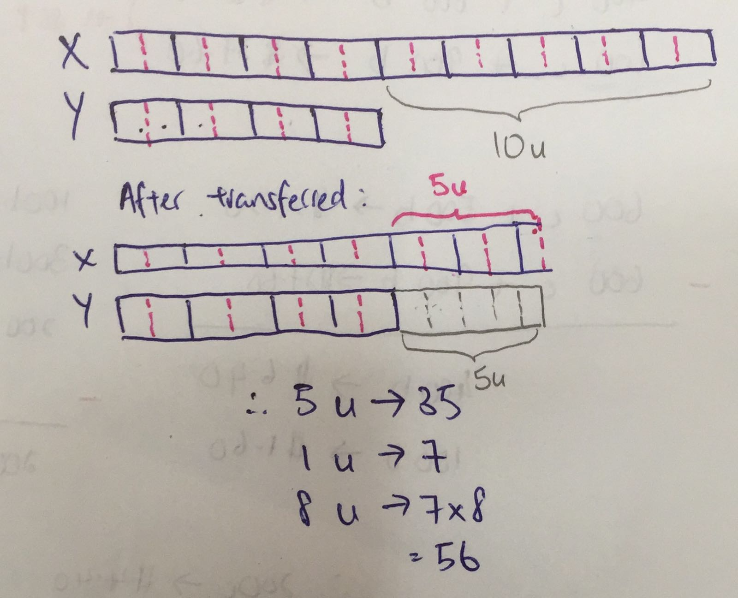# QuestionIf each of us learns how to 1001 games apply and properly study it, everything in the content can be true. Together, let’s build a thriving new world. Many thanks to the banks.

0 Replies 0 Likes

I’m really glad to find the amazing help for the students and they can avail the solution of the assignment easily from an expert. I admire your tremendous btec assignments sharing that is quite supportive to all. Please do share such related material always with us.

0 Replies 0 Likes0 Replies 1 Like

Let the original number of goats in Farm X be x.

Let the original number of goats in Farm Y be y.

Let the final number of goats in Farm X and Y be z

x/y = 9/4

4x = 9y

x – 35 = z

y+35 = z

4(x-35) = 4z

4x – 140 = 4z

9y – 140 = 4z

4(y+35) = 4z

4y + 140 = 4z

9y  – 4y – 140 – 140 = 0

5y – 280 = 0

5y = 280

y =  56